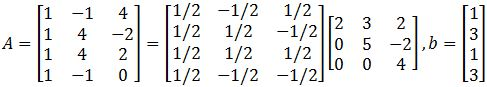# Least-squares problem

### Least-squares problem

#### Lessons

In linear algebra, we have dealt with questions in which $Ax=b$ does not have a solution. When a solution does not exist, the best thing we can do is to approximate $x$. In this section, we will learn how to find a $x$ such that it makes $Ax$ as close as possible to $b$.

If $A$ is an $m \times n$ matrix and $b$ is a vector in $\Bbb{R}^n$, then a least-squares solution of $Ax=b$ is a $\hat{x}$ in $\Bbb{R}^n$ where
$\lVert b-A \hat{x}\rVert \leq \lVert b-Ax\rVert$

For all $x$ in $\Bbb{R}^n$.

The smaller the distance, the smaller the error. Thus, the better the approximation. So the smallest distance gives the best approximation for $x$. So we call the best approximation for $x$ to be $\hat{x}$.

The Least-Squares Solution

The set of least-square solutions of $Ax=b$ matches with the non-empty set of solutions of the matrix equation $A^T A \hat{x}=A^T b$.

In other words,
$A^T A \hat{x}=A^T b$
$\hat{x} = (A^TA)^{-1}A^Tb$

Where $x$ is the least square solutions of $Ax=b$.

Keep in mind that $x$ is not always a unique solution. However, it is unique if one of the conditions hold:
1. The equation $Ax=b$ has unique least-squares solution for each b in $\Bbb{R}^m$.
2. The columns of $A$ are linearly independent.
3. The matrix $A^T A$ is invertible.

The Least-Squares Error
To find the least-squares error of the least-squares solution of $Ax=b$, we compute

$\lVert b - A \hat{x} \rVert$

Alternative Calculations to Least-Squares Solutions
Let $A$ be a $m \times n$ matrix where $a_1,\cdots,a_n$ are the columns of $A$. If $Col(A)=${$a_1,\cdots,a_n$} form an orthogonal set, then we can find the least-squares solutions using the equation
$A \hat{x}=\hat{b}$

where $\hat{b}=proj_{Col(A)}b.$

Let $A$ be a $m \times n$ matrix with linearly independent columns, and let $A=QR$ be the $QR$ factorization of $A$. Then for each $b$ in $\Bbb{R}^m$, the equation $Ax=b$ has a unique least-squares solution where
$\hat{x}=R^{-1} Q^T b$
$R\hat{x}=Q^T b$
• Introduction
Least Squares Problem Overview:
a)
The Least Squares Solution
$Ax=b$ give no solution
• Approximate closest solution $\hat{x}$
• The least Squares Solution $\hat{x} =(A^T A)^{-1} A^T b$
• Not always a unique solution

b)
The Least Squares Error
• Finding the error of the solution $\hat{x}$
• Use the formula $\lVert b-A\hat{x}\rVert$

c)
Alternative Calculation to Least-Squares Solutions
• Orthogonal columns of $A$$A\hat{x} =\hat{b}$
• QR factorization $A=QR$$R\hat{x} =Q^T b$

• 1.
Finding the Least Squares Solutions with $A^T A\hat{x} =A^T b$
Find a least-squares solution of $Ax=b$ if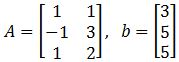• 2.
Describe all least-squares solutions of equation $Ax=b$ if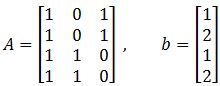• 3.
Finding the Least Squares Error
You are given that the least-squares solution of $Ax=b$ is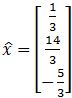. Compute the least-square error if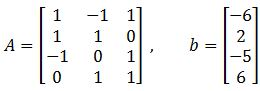• 4.
Finding the Least Squares Solutions with Alternative Ways
Find the orthogonal projections of $b$ onto the columns of $A$ and find a least-squares solution of $Ax=b$.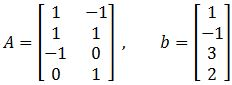• 5.
Use the factorization $A=QR$ to find the least-squares solution of $Ax=b$